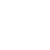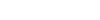## Finance Globe

U.S. financial and economic topics from several finance writers.
Font size: +
2 minutes reading time (312 words)

# How to Calculate Your Monthly Interest Payment

​If you are not a math wizard, and you do not have any kind of accounting / finance background, you may not know how to calculate your monthly interest payment. Yet I can guarantee you will have to pay a monthly interest payment throughout your life. Whether it is through a mortgage, a car loan, a student loan, or a personal loan, you will have to pay some kind of interest payment. I think it is important to be able to at least know how your monthly interest payment is calculated. Below is a high level step by step summary of how to calculate a monthly interest payment.

• The first thing to know is the interest rate is usually quoted as an annual rate. Also, the loan balance will typically change each month so your total payment may change over time or the portion of your payment that goes towards your principal will change over time.
• To figure out your monthly interest rate you will need to divide your annual interest rate by 12 (for the 12 months in the year). As an example, if your annual interest rate is 12%, converting the interest rate to monthly would result in a 1% monthly interest rate (12% divided by 12).
• Next, to calculate the monthly interest payment, you simple multiply your outstanding loan balance by the monthly rate. As an example, if your balance is \$100, then your monthly interest payment would be \$1 (\$100 multiplied by 1%).
• It is a very easy calculation, but if you did not know, now you know how much interest you are paying each month for a given balance. The math can get slightly more complicated if the bank calculates your monthly interest rate by number of days (annual rate divided by 360 multiplied by 30), but the calculation is the same just with more complicated numbers.